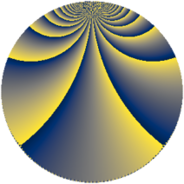# Properties

 Label 1216.4.bjLevel $1216$ Weight $4$ Character orbit 1216.bj Rep. character $\chi_{1216}(161,\cdot)$ Character field $\Q(\zeta_{18})$ Dimension $720$ Sturm bound $640$

# Related objects

## Defining parameters

 Level: $$N$$ $$=$$ $$1216 = 2^{6} \cdot 19$$ Weight: $$k$$ $$=$$ $$4$$ Character orbit: $$[\chi]$$ $$=$$ 1216.bj (of order $$18$$ and degree $$6$$) Character conductor: $$\operatorname{cond}(\chi)$$ $$=$$ $$152$$ Character field: $$\Q(\zeta_{18})$$ Sturm bound: $$640$$

## Dimensions

The following table gives the dimensions of various subspaces of $$M_{4}(1216, [\chi])$$.

Total New Old
Modular forms 2952 720 2232
Cusp forms 2808 720 2088
Eisenstein series 144 0 144

## Trace form

 $$720q + O(q^{10})$$ $$720q - 360q^{41} - 17640q^{49} - 1296q^{73} - 8280q^{81} + O(q^{100})$$

## Decomposition of $$S_{4}^{\mathrm{new}}(1216, [\chi])$$ into newform subspaces

The newforms in this space have not yet been added to the LMFDB.

## Decomposition of $$S_{4}^{\mathrm{old}}(1216, [\chi])$$ into lower level spaces

$$S_{4}^{\mathrm{old}}(1216, [\chi]) \cong$$ $$S_{4}^{\mathrm{new}}(152, [\chi])$$$$^{\oplus 4}$$$$\oplus$$$$S_{4}^{\mathrm{new}}(608, [\chi])$$$$^{\oplus 2}$$